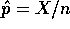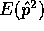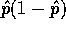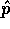STAT 330: 95-3

Midterm, 20 October 1995Instructor: Richard Lockhart

Instructions: This is an open book test. You may use notes, text, other books and a calculator. Your presentations of statistical analysis will be marked for clarity of explanation. I expect you to explain what assumptions you are making and to comment if those assumptions seem unreasonable. The exam is out of 25.

1. A simple random sample of 900 adult Quebec voters is drawn. Each is asked how he or she will vote on the referendum.

1. If 465 say they will vote no and 435 say they will vote yes how strong is the evidence that the referendum will fail? [3 marks]
2. Suppose that a week earlier a survey of 1200 voters had given 650 yes and 550 no. Has the proportion of the population who will vote no increased? [3 marks]
3. Briefly (2 or 3 sentences) criticize the assumptions used in the previous two parts and comment on the realism of the description of outcome of the survey. [1 mark]

2. Government offices maintain standard weights, whose weights are known very accurately, in order to compare other weights and keep scales well standardized. One of these weights is weighed 10 times giving an average of 496 micrograms over 10 grams; the SD of these measurements is 30 micrograms.

1. Give a 90% confidence interval for the true weight explaining your assumptions. [5 marks]
2. The weight is then dropped accidentally and weighed 6 further times, averaging 465 micrograms over 10 grams with an SD of 25 micrograms. Did the weight change when it was dropped? [5 marks]
3. Assume that the scales make errors with an SD of 30 micrograms when weighing objects whose true weight is close to 10 grams. How many times would you have to weigh such a weight to have at least a 95% chance of detecting a difference of 5 micrograms from 10 grams, using a 10% level test. [3 marks]
3. Consider a factory producing a very large number of bolts each day. A sample of n of these bolts is drawn, each is tested for strength and a total of X of these bolts fail the test. Let p be the fraction of the day's production which would fail the test (if tested). Letbe the sample estimate of p.

1. Find. [2 marks]
2. Consider the estimatorof p(1-p). What is the bias of this estimator? [2 marks]
3. What is the maximum likelihood estimator of p(1-p)? (You may use the fact thatis the maximum likelihood estimator of p.) [1 mark]

Richard Lockhart
Wed Feb 11 09:08:35 PST 1998